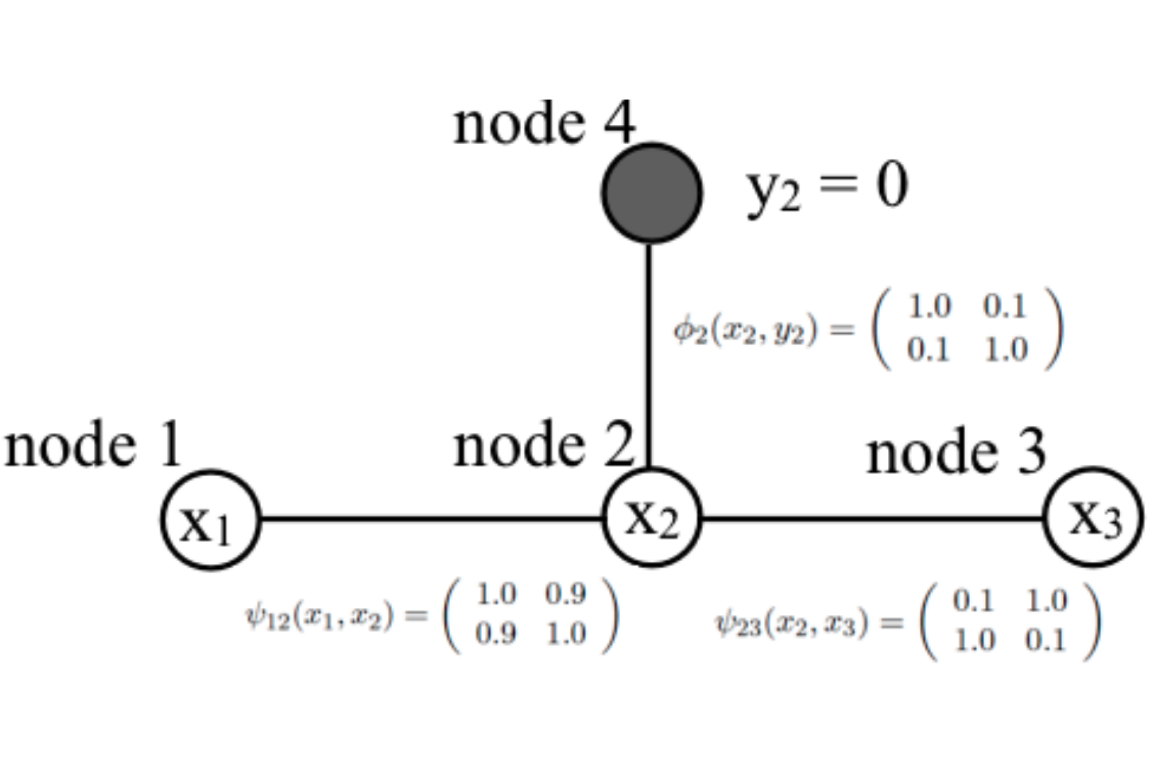October 4, 2016

## Lecture 8 Main Points Once Again

• Belief propagation (Pearl 1988; Lauritzen and Spiegelhalter, 1988, JRSS-B)
• Computes the exact marginal probability for each node in a factor graph that has a tree structure
• Reduced computational complexity. For example, in a chain graph with $$d$$ discrete variables ($$k$$ states), from exponential ($$\mathcal{O}(k^d)$$) to linear in the number of nodes and quardratic in the number of states ($$\mathcal{O}(dk^2)$$, why?)
• No reduction in computational complexity of exact inference if the graph is fully connected: we are forced to work with the full joint distribution
• Share computations efficiently when many marginal probabilities are required (example on whiteboard)
• In belief propagation for finding the marginal probability at every node, a message is a re-usable partial sum for the marginalization calculations.

## Numerical Example of Belief Propagation (Freeman and Torralba)

• Example: tree-structured undirected graph with pairwise potentials
• Binary variables $$x_j$$, $$j=1,2,3$$ (unobserved), and $$y_2=0$$ (observed).
• Calculate $$p(x_j=0), j=1,2,3$$, using messages between nodes (whiteboard)## Factor Graphs as a Tool to Organize Messages

Remark:

• Any factor graph could be transformed to an undirected graph with pairwise potentials (Yedidia et al. 2003). The previous example demonstrates a general message-passing strategy on undirected graphs with pairwise potentials.
• Any undirected graphs or directed acyclic graphs can be transformed to factor graphs

## Notations

• $$X$$: single variable node; $$x$$: an instance of $$X$$
• $$\mathbf{X}$$: a vector of variable nodes, e.g., $$\mathbf{X}=(X_1,X_2,X_3)'$$; $$\mathbf{x}$$ (e.g., $$\mathbf{x}=(x_1,x_2,x_3)'$$): an instance of the random vector $$\mathbf{X}$$
• $$f_C$$: a factor/potential in the factorization of the joint distribution: $p(\mathbf{X}=\mathbf{x})\propto \prod_{C: \text{cliques in an undirected graph \mathcal{H}}}f_C(\mathbf{X}_C),$ where a clique $$C$$ collects the relevant nodes, say, $$C=\{2,3\}$$ and $$\mathbf{X}_C=(X_2,X_3)$$ denotes the vector of variables nodes involved in Clique $$C$$.
• $$\psi_C$$: same as $$f_C$$ (used interchangeably in the class)
• $$N(X)$$ or $$N(f)$$: the neighbor of a variable node or a factor node.

## Sum-Product Algorithm for Factor Trees

The marginal probability $$p(X_i=x_i)=\prod_{f_C\in N(X_i)} m_{f_C\rightarrow X_i}(x_i)$$. Choose a variable node as the root ($$X_R$$), then starting from the leaves:

1. If $$f_C$$ is a leaf node, then $$m_{f_C\rightarrow X}(x_C)=f_C(x_C)$$
2. If $$X$$ is a leaf node, then $$m_{X\rightarrow f_C}(x)=1$$
3. The message sent from a non-leaf factor node $$f_C$$ to a variable node $$X_i$$ is $m_{f_C\rightarrow X_i}(x_i)=\sum_{x_{N(f_C)-X_i}}f_C(\mathbf{x}_C)\prod_{x': X'\in {N(f_C)-X_i}} m_{X'\rightarrow f_C}(x')$
4. The message sent from a non-leaf variable node $$X_i$$ to a factor node $$f_C$$ is: $m_{X_i\rightarrow f_C}(x_i) =\prod_{f_{C'} \in N(X_i)-f_C} m_{f_{C'}\rightarrow X_i}(x_i)$

## Remarks: Sum-Product Algorithm

• "A factor collects messages sent to its arms (except the destination arm), bundles them with its direct interaction with the destination variable node, and collapses (integrates over) the arms (except the destination arm)."
• "A variable node bundles (mutiplies) the incoming messages and sends it to the destination factor node"
• In Step 2, a leaf variable node has no extra information to bundle, just equally weight all $$X=x$$ (recall that messages form a vector of dimension $$|X|$$, each corresponding to a value of $$x$$)
• In Step 4, when a variable node has only two factor neighbors, the rule means: "$$X_i$$, please just pass the message from factor $$f_{C'}$$ to $$f_{C}$$, do nothing else!"
• In Step 3, to find the messages from a factor $$f_C$$ to $$X_i$$, we integrate all the uncertainties/information about variables other than $$X_i$$ but pertaining to $$f_C$$. We multiply $$f_C$$ by other messages sent to its neighboring nodes (except $$X_i$$)